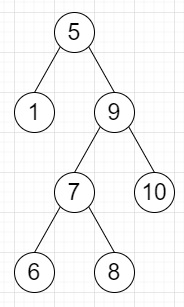# Program to check whether a binary tree is BST or not in Python

Suppose we have binary tree; we have to check whether it is a binary search tree or not. As we know a BST has following properties −

• all nodes on its left subtree is smaller than current node value
• all nodes on its right subtree is larger than current node value
• these properties hold recursively for all nodes

So, if the input is likethen the output will be True

To solve this, we will follow these steps −

• x := a list of inorder traversal sequence of tree elements
• if x is sorted, then
• return true
• return false

Let us see the following implementation to get better understanding −

## Example

Live Demo

class TreeNode:
def __init__(self, data, left = None, right = None):
self.data = data
self.left = left
self.right = right
class Solution:
def solve(self, root):
def inorder(root,l):
if root is None:
return
inorder(root.left,l) l.append(root.data)
inorder(root.right,l)
l = []
inorder(root,l)
return l == sorted(l)
ob = Solution()
root = TreeNode(5)
root.left = TreeNode(1)
root.right = TreeNode(9) root.right.left = TreeNode(7) root.right.right = TreeNode(10) root.right.left.left = TreeNode(6) root.right.left.right = TreeNode(8) print(ob.solve(root))

## Input

root = TreeNode(5)
root.left = TreeNode(1)
root.right = TreeNode(9)
root.right.left = TreeNode(7)
root.right.right = TreeNode(10)
root.right.left.left = TreeNode(6)
root.right.left.right = TreeNode(8)

## Output

True

Updated on: 06-Oct-2020

458 Views